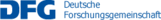B04 – Random matrices and random surfaces

In the last 10 years a lot of progress has been achieved both in the field of random matrices and for random surfaces in the Kardar-Parisi-Zhang (KPZ) universality class. In random matrices, the central questions of universality in the limit of large matrix size, both in the bulk and at the edge of the spectrum, have been solved for a wide class of matrices (with independent entries or basis invariance). Unlike the first results in the field, where one used the knowledge of the correlation functions to answer the questions, the methods recently used are much more robust. For growth models belonging to the KPZ class, the first universality results (showing up at large growth time) were also obtained using the information on correlation functions. Recently, there have been results for models which do not have determinantal correlation functions, like the KPZ equation itself, the asymmetric exclusion process, or a directed polymer model at non-zero temperature. However, the degree of universality results achieved in random matrix theory is not yet achieved for KPZ growth models.

In this project we pursue the goal of showing universality but we also focus on the convergence to universality, i.e., the non-asymptotic regimes. For random surfaces arising from growth models, we will in particular consider systems where the correlation functions are not explicitly known. Also, we wish to better understand the link to random matrices. For that reason, it is important to answer the question of universality for the minor process of random matrices (which is the process describing the joint eigenvalues of a matrix and its main minors), both at the edge and in the bulk of the spectrum. Indeed, several of the recently solved models in the KPZ class have a hidden structure similar to the Gelfand-Tseltin scheme present for the minor process. Non-asymptotic results for the minor process might be useful to answer a question of the Project C02 too.

Name Institute Location Phone
Ferrari, Patrik IAM En60/3.047 2747 ferrari@uni-bonn.de
Peltola, Eveliina IAM En60/3.042 3172 eveliina.peltola@hcm.uni-bonn.de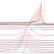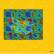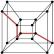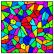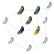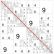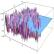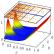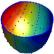Contact

Coordinator: Prof. Dr. Stefan Müller